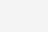# 八大排序算法

## 概述当n较大，则应采用时间复杂度为O(nlog2n)的排序方法：快速排序、堆排序或归并排序序。

快速排序：是目前基于比较的内部排序中被认为是最好的方法，当待排序的关键字是随机分布时，快速排序的平均时间最短；

## 1.插入排序—直接插入排序(Straight Insertion Sort)```void print(int a[], int n ,int i){
cout<<i <<":";
for(int j= 0; j<8; j++){
cout<<a[j] <<" ";
}
cout<<endl;
}

void InsertSort(int a[], int n)
{
for(int i= 1; i<n; i++){
if(a[i] < a[i-1]){               //若第i个元素大于i-1元素，直接插入。小于的话，移动有序表后插入
int j= i-1;
int x = a[i];        //复制为哨兵，即存储待排序元素
a[i] = a[i-1];           //先后移一个元素
while(x < a[j]){  //查找在有序表的插入位置
a[j+1] = a[j];
j--;         //元素后移
}
a[j+1] = x;      //插入到正确位置
}
print(a,n,i);           //打印每趟排序的结果
}

}

int main(){
int a = {3,1,5,7,2,4,9,6};
InsertSort(a,8);
print(a,8,8);
}```

## 2. 插入排序—希尔排序（Shell`s Sort）

1. 选择一个增量序列t1，t2，…，tk，其中ti>tj，tk=1；

2. 按增量序列个数k，对序列进行k 趟排序；

3. 每趟排序，根据对应的增量ti，将待排序列分割成若干长度为m 的子序列，分别对各子表进行直接插入排序。仅增量因子为1 时，整个序列作为一个表来处理，表长度即为整个序列的长度。```void print(int a[], int n ,int i){
cout<<i <<":";
for(int j= 0; j<8; j++){
cout<<a[j] <<" ";
}
cout<<endl;
}
/**
* 直接插入排序的一般形式
*
* @param int dk 缩小增量，如果是直接插入排序，dk=1
*
*/

void ShellInsertSort(int a[], int n, int dk)
{
for(int i= dk; i<n; ++i){
if(a[i] < a[i-dk]){          //若第i个元素大于i-1元素，直接插入。小于的话，移动有序表后插入
int j = i-dk;
int x = a[i];           //复制为哨兵，即存储待排序元素
a[i] = a[i-dk];         //首先后移一个元素
while(x < a[j]){     //查找在有序表的插入位置
a[j+dk] = a[j];
j -= dk;             //元素后移
}
a[j+dk] = x;            //插入到正确位置
}
print(a, n,i );
}

}

/**
* 先按增量d（n/2,n为要排序数的个数进行希尔排序
*
*/
void shellSort(int a[], int n){

int dk = n/2;
while( dk >= 1  ){
ShellInsertSort(a, n, dk);
dk = dk/2;
}
}
int main(){
int a = {3,1,5,7,2,4,9,6};
//ShellInsertSort(a,8,1); //直接插入排序
shellSort(a,8);           //希尔插入排序
print(a,8,8);
}```

## 3. 选择排序—简单选择排序（Simple Selection Sort）```void print(int a[], int n ,int i){
cout<<"第"<<i+1 <<"趟 : ";
for(int j= 0; j<8; j++){
cout<<a[j] <<"  ";
}
cout<<endl;
}
/**
* 数组的最小值
*
* @return int 数组的键值
*/
int SelectMinKey(int a[], int n, int i)
{
int k = i;
for(int j=i+1 ;j< n; ++j) {
if(a[k] > a[j]) k = j;
}
return k;
}

/**
* 选择排序
*
*/
void selectSort(int a[], int n){
int key, tmp;
for(int i = 0; i< n; ++i) {
key = SelectMinKey(a, n,i);           //选择最小的元素
if(key != i){
tmp = a[i];  a[i] = a[key]; a[key] = tmp; //最小元素与第i位置元素互换
}
print(a,  n , i);
}
}
int main(){
int a = {3,1,5,7,2,4,9,6};
cout<<"初始值：";
for(int j= 0; j<8; j++){
cout<<a[j] <<"  ";
}
cout<<endl<<endl;
selectSort(a, 8);
print(a,8,8);
}```

简单选择排序的改进——二元选择排序

```void SelectSort(int r[],int n) {
int i ,j , min ,max, tmp;
for (i=1 ;i <= n/2;i++) {
// 做不超过n/2趟选择排序
min = i; max = i ; //分别记录最大和最小关键字记录位置
for (j= i+1; j<= n-i; j++) {
if (r[j] > r[max]) {
max = j ; continue ;
}
if (r[j]< r[min]) {
min = j ;
}
}
//该交换操作还可分情况讨论以提高效率
tmp = r[i-1]; r[i-1] = r[min]; r[min] = tmp;
tmp = r[n-i]; r[n-i] = r[max]; r[max] = tmp;

}
}```

## 4. 选择排序—堆排序（Heap Sort）（a）大顶堆序列：（96, 83,27,38,11,09)

(b)  小顶堆序列：（12，36，24，85，47，30，53，91）1. 如何将n 个待排序的数建成堆；
2. 输出堆顶元素后，怎样调整剩余n-1 个元素，使其成为一个新堆。

1）设有m 个元素的堆，输出堆顶元素后，剩下m-1 个元素。将堆底元素送入堆顶（（最后一个元素与堆顶进行交换），堆被破坏，其原因仅是根结点不满足堆的性质。

2）将根结点与左、右子树中较小元素的进行交换。

3）若与左子树交换：如果左子树堆被破坏，即左子树的根结点不满足堆的性质，则重复方法 （2）.

4）若与右子树交换，如果右子树堆被破坏，即右子树的根结点不满足堆的性质。则重复方法 （2）.

5）继续对不满足堆性质的子树进行上述交换操作，直到叶子结点，堆被建成。1）n 个结点的完全二叉树，则最后一个结点是第个结点的子树。

2）筛选从第个结点为根的子树开始，该子树成为堆。

3）之后向前依次对各结点为根的子树进行筛选，使之成为堆，直到根结点。算法的实现：

```void print(int a[], int n){
for(int j= 0; j<n; j++){
cout<<a[j] <<"  ";
}
cout<<endl;
}

/**
* 已知H[s…m]除了H[s] 外均满足堆的定义
* 调整H[s],使其成为大顶堆.即将对第s个结点为根的子树筛选,
*
* @param H是待调整的堆数组
* @param s是待调整的数组元素的位置
* @param length是数组的长度
*
*/
void HeapAdjust(int H[],int s, int length)
{
int tmp  = H[s];
int child = 2*s+1; //左孩子结点的位置。(i+1 为当前调整结点的右孩子结点的位置)
while (child < length) {
if(child+1 <length && H[child]<H[child+1]) { // 如果右孩子大于左孩子(找到比当前待调整结点大的孩子结点)
++child ;
}
if(H[s]<H[child]) {  // 如果较大的子结点大于父结点
H[s] = H[child]; // 那么把较大的子结点往上移动，替换它的父结点
s = child;       // 重新设置s ,即待调整的下一个结点的位置
child = 2*s+1;
}  else {            // 如果当前待调整结点大于它的左右孩子，则不需要调整，直接退出
break;
}
H[s] = tmp;         // 当前待调整的结点放到比其大的孩子结点位置上
}
print(H,length);
}

/**
* 初始堆进行调整
* 将H[0..length-1]建成堆
* 调整完之后第一个元素是序列的最小的元素
*/
void BuildingHeap(int H[], int length)
{
//最后一个有孩子的节点的位置 i=  (length -1) / 2
for (int i = (length -1) / 2 ; i >= 0; --i)
}
/**
* 堆排序算法
*/
void HeapSort(int H[],int length)
{
//初始堆
BuildingHeap(H, length);
//从最后一个元素开始对序列进行调整
for (int i = length - 1; i > 0; --i)
{
//交换堆顶元素H和堆中最后一个元素
int temp = H[i]; H[i] = H; H = temp;
//每次交换堆顶元素和堆中最后一个元素之后，都要对堆进行调整
}
}

int main(){
int H = {3,1,5,7,2,4,9,6,10,8};
cout<<"初始值：";
print(H,10);
HeapSort(H,10);
//selectSort(a, 8);
cout<<"结果：";
print(H,10);

}```## 5. 交换排序—冒泡排序（Bubble Sort）```void bubbleSort(int a[], int n){
for(int i =0 ; i< n-1; ++i) {
for(int j = 0; j < n-i-1; ++j) {
if(a[j] > a[j+1])
{
int tmp = a[j] ; a[j] = a[j+1] ;  a[j+1] = tmp;
}
}
}
}```

1．设置一标志性变量pos,用于记录每趟排序中最后一次进行交换的位置。由于pos位置之后的记录均已交换到位,故在进行下一趟排序时只要扫描到pos位置即可。

```void Bubble_1 ( int r[], int n) {
int i= n -1;  //初始时,最后位置保持不变
while ( i> 0) {
int pos= 0; //每趟开始时,无记录交换
for (int j= 0; j< i; j++)
if (r[j]> r[j+1]) {
pos= j; //记录交换的位置
int tmp = r[j]; r[j]=r[j+1];r[j+1]=tmp;
}
i= pos; //为下一趟排序作准备
}
}```

2．传统冒泡排序中每一趟排序操作只能找到一个最大值或最小值,我们考虑利用在每趟排序中进行正向和反向两遍冒泡的方法一次可以得到两个最终值(最大者和最小者) , 从而使排序趟数几乎减少了一半。

```void Bubble_2 ( int r[], int n){
int low = 0;
int high= n -1; //设置变量的初始值
int tmp,j;
while (low < high) {
for (j= low; j< high; ++j) //正向冒泡,找到最大者
if (r[j]> r[j+1]) {
tmp = r[j]; r[j]=r[j+1];r[j+1]=tmp;
}
--high;                 //修改high值, 前移一位
for ( j=high; j>low; --j) //反向冒泡,找到最小者
if (r[j]<r[j-1]) {
tmp = r[j]; r[j]=r[j-1];r[j-1]=tmp;
}
++low;                  //修改low值,后移一位
}
}```

## 6. 交换排序—快速排序（Quick Sort）

1）选择一个基准元素,通常选择第一个元素或者最后一个元素,

2）通过一趟排序讲待排序的记录分割成独立的两部分，其中一部分记录的元素值均比基准元素值小。另一部分记录的 元素值比基准值大。

3）此时基准元素在其排好序后的正确位置

4）然后分别对这两部分记录用同样的方法继续进行排序，直到整个序列有序。

（a）一趟排序的过程：（b）排序的全过程递归实现：

```void print(int a[], int n){
for(int j= 0; j<n; j++){
cout<<a[j] <<"  ";
}
cout<<endl;
}

void swap(int *a, int *b)
{
int tmp = *a;
*a = *b;
*b = tmp;
}

int partition(int a[], int low, int high)
{
int privotKey = a[low];                             //基准元素
while(low < high){                                   //从表的两端交替地向中间扫描
while(low < high  && a[high] >= privotKey) --high;  //从high 所指位置向前搜索，至多到low+1 位置。将比基准元素小的交换到低端
swap(&a[low], &a[high]);
while(low < high  && a[low] <= privotKey ) ++low;
swap(&a[low], &a[high]);
}
print(a,10);
return low;
}

void quickSort(int a[], int low, int high){
if(low < high){
int privotLoc = partition(a,  low,  high);  //将表一分为二
quickSort(a,  low,  privotLoc -1);          //递归对低子表递归排序
quickSort(a,   privotLoc + 1, high);        //递归对高子表递归排序
}
}

int main(){
int a = {3,1,5,7,2,4,9,6,10,8};
cout<<"初始值：";
print(a,10);
quickSort(a,0,9);
cout<<"结果：";
print(a,10);

}```

```void print(int a[], int n){
for(int j= 0; j<n; j++){
cout<<a[j] <<"  ";
}
cout<<endl;
}

void swap(int *a, int *b)
{
int tmp = *a;
*a = *b;
*b = tmp;
}

int partition(int a[], int low, int high)
{
int privotKey = a[low];                 //基准元素
while(low < high){                   //从表的两端交替地向中间扫描
while(low < high  && a[high] >= privotKey) --high; //从high 所指位置向前搜索，至多到low+1 位置。将比基准元素小的交换到低端
swap(&a[low], &a[high]);
while(low < high  && a[low] <= privotKey ) ++low;
swap(&a[low], &a[high]);
}
print(a,10);
return low;
}

void qsort_improve(int r[ ],int low,int high, int k){
if( high -low > k ) { //长度大于k时递归, k为指定的数
int pivot = partition(r, low, high); // 调用的Partition算法保持不变
qsort_improve(r, low, pivot - 1,k);
qsort_improve(r, pivot + 1, high,k);
}
}
void quickSort(int r[], int n, int k){
qsort_improve(r,0,n,k);//先调用改进算法Qsort使之基本有序

//再用插入排序对基本有序序列排序
for(int i=1; i<=n;i ++){
int tmp = r[i];
int j=i-1;
while(tmp < r[j]){
r[j+1]=r[j]; j=j-1;
}
r[j+1] = tmp;
}

}

int main(){
int a = {3,1,5,7,2,4,9,6,10,8};
cout<<"初始值：";
print(a,10);
quickSort(a,9,4);
cout<<"结果：";
print(a,10);

}```

## 7. 归并排序（Merge Sort）1. j=m+1；k=i；i=i; //置两个子表的起始下标及辅助数组的起始下标

2. 若i>m 或j>n，转⑷ //其中一个子表已合并完，比较选取结束

3. //选取r[i]和r[j]较小的存入辅助数组rf
如果r[i]<r[j]，rf[k]=r[i]； i++； k++； 转⑵
否则，rf[k]=r[j]； j++； k++； 转⑵

4. //将尚未处理完的子表中元素存入rf
如果i<=m，将r[i…m]存入rf[k…n] //前一子表非空
如果j<=n ,  将r[j…n] 存入rf[k…n] //后一子表非空

5. 合并结束。

```//将r[i…m]和r[m +1 …n]归并到辅助数组rf[i…n]
void Merge(ElemType *r,ElemType *rf, int i, int m, int n)
{
int j,k;
for(j=m+1,k=i; i<=m && j <=n ; ++k){
if(r[j] < r[i]) rf[k] = r[j++];
else rf[k] = r[i++];
}
while(i <= m)  rf[k++] = r[i++];
while(j <= n)  rf[k++] = r[j++];
}```

1 个元素的表总是有序的。所以对n 个元素的待排序列，每个元素可看成1 个有序子表。对子表两两合并生成n/2个子表，所得子表除最后一个子表长度可能为1 外，其余子表长度均为2。再进行两两合并，直到生成n 个元素按关键码有序的表。

```void print(int a[], int n){
for(int j= 0; j<n; j++){
cout<<a[j] <<"  ";
}
cout<<endl;
}

//将r[i…m]和r[m +1 …n]归并到辅助数组rf[i…n]
void Merge(ElemType *r,ElemType *rf, int i, int m, int n)
{
int j,k;
for(j=m+1,k=i; i<=m && j <=n ; ++k){
if(r[j] < r[i]) rf[k] = r[j++];
else rf[k] = r[i++];
}
while(i <= m)  rf[k++] = r[i++];
while(j <= n)  rf[k++] = r[j++];
print(rf,n+1);
}

void MergeSort(ElemType *r, ElemType *rf, int lenght)
{
int len = 1;
ElemType *q = r ;
ElemType *tmp ;
while(len < lenght) {
int s = len;
len = 2 * s ;
int i = 0;
while(i+ len <lenght){
Merge(q, rf,  i, i+ s-1, i+ len-1 ); //对等长的两个子表合并
i = i+ len;
}
if(i + s < lenght){
Merge(q, rf,  i, i+ s -1, lenght -1); //对不等长的两个子表合并
}
tmp = q; q = rf; rf = tmp; //交换q,rf，以保证下一趟归并时，仍从q 归并到rf
}
}

int main(){
int a = {3,1,5,7,2,4,9,6,10,8};
int b;
MergeSort(a, b, 10);
print(b,10);
cout<<"结果：";
print(a,10);

}```

```void MSort(ElemType *r, ElemType *rf,int s, int t)
{
ElemType *rf2;
if(s==t) r[s] = rf[s];
else
{
int m=(s+t)/2;          /*平分*p 表*/
MSort(r, rf2, s, m);        /*递归地将p[s…m]归并为有序的p2[s…m]*/
MSort(r, rf2, m+1, t);      /*递归地将p[m+1…t]归并为有序的p2[m+1…t]*/
Merge(rf2, rf, s, m+1,t);   /*将p2[s…m]和p2[m+1…t]归并到p1[s…t]*/
}
}
void MergeSort_recursive(ElemType *r, ElemType *rf, int n)
{   /*对顺序表*p 作归并排序*/
MSort(r, rf,0, n-1);
}```

简单来说，就是把数据分组，放在一个个的桶中，然后对每个桶里面的在进行排序。

例如要对大小为[1..1000]范围内的n个整数A[1..n]排序

首先，可以把桶设为大小为10的范围，具体而言，设集合B存储[1..10]的整数，集合B存储   (10..20]的整数，……集合B[i]存储(   (i-1)*10,   i*10]的整数，i   =   1,2,..100。总共有  100个桶。

然后，对A[1..n]从头到尾扫描一遍，把每个A[i]放入对应的桶B[j]中。  再对这100个桶中每个桶里的数字排序，这时可用冒泡，选择，乃至快排，一般来说任  何排序法都可以。

最后，依次输出每个桶里面的数字，且每个桶中的数字从小到大输出，这  样就得到所有数字排好序的一个序列了。

假设有n个数字，有m个桶，如果数字是平均分布的，则每个桶里面平均有n/m个数字。如果

对每个桶中的数字采用快速排序，那么整个算法的复杂度是

O(n   +   m   *   n/m*log(n/m))   =   O(n   +   nlogn   –   nlogm)

从上式看出，当m接近n的时候，桶排序复杂度接近O(n)

当然，以上复杂度的计算是基于输入的n个数字是平均分布这个假设的。这个假设是很强的  ，实际应用中效果并没有这么好。如果所有的数字都落在同一个桶中，那就退化成一般的排序了。

前面说的几大排序算法 ，大部分时间复杂度都是O（n2），也有部分排序算法时间复杂度是O(nlogn)。而桶式排序却能实现O（n）的时间复杂度。但桶排序的缺点是：

1）首先是空间复杂度比较高，需要的额外开销大。排序有两个数组的空间开销，一个存放待排序数组，一个就是所谓的桶，比如待排序值是从0到m-1，那就需要m个桶，这个桶数组就要至少m个空间。

2）其次待排序的元素都要在一定的范围内等等。

桶式排序是一种分配排序。分配排序的特定是不需要进行关键码的比较，但前提是要知道待排序列的一些具体情况。1）先按k1 排序分组，将序列分成若干子序列，同一组序列的记录中，关键码k1 相等。

2）再对各组按k2 排序分成子组，之后，对后面的关键码继续这样的排序分组，直到按最次位关键码kd 对各子组排序后。

3）再将各组连接起来，便得到一个有序序列。扑克牌按花色、面值排序中介绍的方法一即是MSD 法。

1) 先从kd 开始排序，再对kd-1进行排序，依次重复，直到按k1排序分组分成最小的子序列后。

2) 最后将各个子序列连接起来，便可得到一个有序的序列, 扑克牌按花色、面值排序中介绍的方法二即是LSD 法。

“多关键字排序”的思想实现“单关键字排序”。对数字型或字符型的单关键字，可以看作由多个数位或多个字符构成的多关键字，此时可以采用“分配-收集”的方法进行排序，这一过程称作基数排序法，其中每个数字或字符可能的取值个数称为基数。比如，扑克牌的花色基数为4，面值基数为13。在整理扑克牌时，既可以先按花色整理，也可以先按面值整理。按花色整理时，先按红、黑、方、花的顺序分成4摞（分配），再按此顺序再叠放在一起（收集），然后按面值的顺序分成13摞（分配），再按此顺序叠放在一起（收集），如此进行二次分配和收集即可将扑克牌排列有序。

```Void RadixSort(Node L[],length,maxradix)
{
int m,n,k,lsp;
k=1;m=1;
int temp[length-1];
Empty(temp); //清空临时空间
{
for(int i=0;i<length;i++) //分配过程
{
if(L[i]<m)
Temp[n]=L[i];
else
Lsp=(L[i]/m)%10; //确定关键字
Temp[lsp][n]=L[i];
n++;
}
CollectElement(L,Temp); //收集
n=0;
m=m*10;
k++;
}
}```

## 总结我们比较时间复杂度函数的情况：时间复杂度函数O(n)的增长情况(1)平方阶(O(n2))排序
各类简单排序:直接插入、直接选择和冒泡排序；
(2)线性对数阶(O(nlog2n))排序
快速排序堆排序归并排序
(3)O(n1+§))排序,§是介于0和1之间的常数。

希尔排序
(4)线性阶(O(n))排序
基数排序，此外还有桶、箱排序。

稳定性的好处：排序算法如果是稳定的，那么从一个键上排序，然后再从另一个键上排序，第一个键排序的结果可以为第二个键排序所用。基数排序就是这样，先按低位排序，逐次按高位排序，低位相同的元素其顺序再高位也相同时是不会改变的。另外，如果排序算法稳定，可以避免多余的比较；

1．待排序的记录数目n的大小；

2．记录本身数据量的大小，也就是记录中除关键字外的其他信息量的大小；

3．关键字的结构及其分布情况；

4．对排序稳定性的要求。

1）当n较大，则应采用时间复杂度为O(nlog2n)的排序方法：快速排序、堆排序或归并排序序。

快速排序：是目前基于比较的内部排序中被认为是最好的方法，当待排序的关键字是随机分布时，快速排序的平均时间最短；
堆排序 ：  如果内存空间允许且要求稳定性的，

归并排序：它有一定数量的数据移动，所以我们可能过与插入排序组合，先获得一定长度的序列，然后再合并，在效率上将有所提高。

2）  当n较大，内存空间允许，且要求稳定性 =》归并排序

3）当n较小，可采用直接插入或直接选择排序。

直接插入排序：当元素分布有序，直接插入排序将大大减少比较次数和移动记录的次数。

直接选择排序 ：元素分布有序，如果不要求稳定性，选择直接选择排序

5）一般不使用或不直接使用传统的冒泡排序。

6）基数排序

1、关键字可分解。

2、记录的关键字位数较少，如果密集更好
3、如果是数字时，最好是无符号的，否则将增加相应的映射复杂度，可先将其正负分开排序。

(0)

### 相关推荐

• #### linux 必须掌握的60个命令

Linux提供了大量的命令，利用它可以有效地完成大量的工 作，如磁盘操作、文件存取、目录操作、进程管理、文件权限设定等。所以，在Linux系统上工作离不开使用系统提供的命令。要想真正理解Linux系统， 就必须从Linux命令学起，通过基础的命令学习可以进一步理解Linux系统。 不同Linux发行版的命令数量不一样，但Linux发行版本最少的命令也有200…

2017-09-05
• #### Linux 文件系统上的权限

文件系统上的权限是指文件系统上的文件和目录的权限，由于Linux是一种多用户的操作系统，而且允许同一时间登录多个用户操作，所以我们就需要一定的管理机制来对限定不同用户对同一文件或目录的操作权限。 文件系统上的权限主要针对三类对象（访问者）进行定义： owner:属主 u; g 属组 group; o: 其它 other 每个文件针对每类访问者都定义了三种权限…

2017-06-04
• #### Cobbler 自动化部署系统

本人较懒，做好笔记，预排版，打印成 PDF 了。-_## Cobbler 自动化部署系统.pdf

Linux干货 2015-08-17
• #### 马哥Linux第六周作业

网络管理 进程查看及管理之ps,top,htop,dstat,vmstat

2018-01-20
• #### Linux基础 sed命令详解

概述 sed是一个流编辑器（Stream EDitor）。主要用于自动编辑一个或多个文件；简化对文件的反复操作；编写转换程序等。本文主要讲述了： sed工作原理 sed命令格式及常用选项 应用实例 高级编辑命令 sed工作原理 sed命令运行过程中维护着两个缓冲区，一个是活动的“模式空间（pattern space）”，另一个是起辅助作用的“暂存缓冲区（ho…

Linux干货 2016-08-12
• #### ☞LAMP

LAMP lamp环境简介 php –> httpd Module形式安装php FastCGI方式安装php httpd-2.2配置fcgi php-xcache MySQL 安装与配置 php –> MySQL 快速部署LAMP php网站程序安装 phpMyadmin wordpress DiscuzX 脚本安装wordpress、disc…

Linux干货 2016-10-12